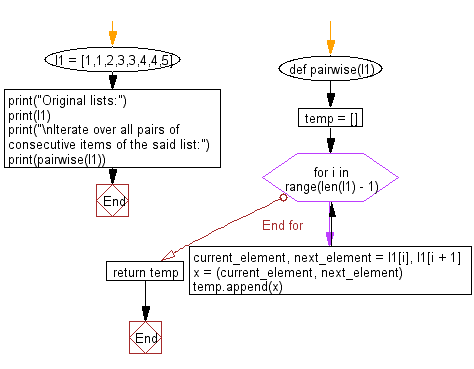﻿ Python: Iterate over all pairs of consecutive items in a given list - w3resource# Python: Iterate over all pairs of consecutive items in a given list

## Python List: Exercise - 135 with Solution

Write a Python program to iterate over all pairs of consecutive items in a given list.

Sample Solution:

Python Code:

``````def pairwise(l1):
temp = []
for i in range(len(l1) - 1):
current_element, next_element = l1[i], l1[i + 1]
x = (current_element, next_element)
temp.append(x)
return temp
l1 = [1,1,2,3,3,4,4,5]
print("Original lists:")
print(l1)
print("\nIterate over all pairs of consecutive items of the said list:")
print(pairwise(l1))
```
```

Sample Output:

```Original lists:
[1, 1, 2, 3, 3, 4, 4, 5]

Iterate over all pairs of consecutive items of the said list:
[(1, 1), (1, 2), (2, 3), (3, 3), (3, 4), (4, 4), (4, 5)]
```

Flowchart:## Visualize Python code execution:

The following tool visualize what the computer is doing step-by-step as it executes the said program:

Python Code Editor:

Have another way to solve this solution? Contribute your code (and comments) through Disqus.

What is the difficulty level of this exercise?

Test your Python skills with w3resource's quiz

﻿

## Python: Tips of the Day

Floor Division:

When we speak of division we normally mean (/) float division operator, this will give a precise result in float format with decimals.

For a rounded integer result there is (//) floor division operator in Python. Floor division will only give integer results that are round numbers.

```print(1000 // 300)
print(1000 / 300)```

Output:

```3
3.3333333333333335```